# Pfaffian equation

(diff) ← Older revision | Latest revision (diff) | Newer revision → (diff)

An equation of the form(1)

where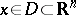,is a differential-form (cf. Differential form), and the functions,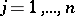, are real-valued. Let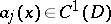and suppose that the vector field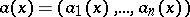does not have critical points in the domain.

A manifold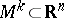of dimension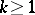and of classis called an integral manifold of the Pfaffian equation (1) if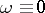on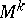. The Pfaffian equation is said to be completely integrable if there is one and only one integral manifold of maximum possible dimensionthrough each point of the domain.

Frobenius' theorem: A necessary and sufficient condition for the Pfaffian equation (1) to be completely integrable is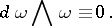(2)

Here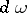is the differential form of degree 2 obtained fromby exterior differentiation, and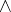is the exterior product. In this case the integration of the Pfaffian equation reduces to the integration of a system of ordinary differential equations.

In three-dimensional Euclidean space a Pfaffian equation has the form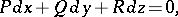(3)

where,andare functions of,andand condition (2) for complete integrability is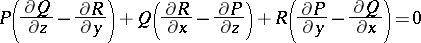(4)

or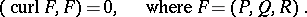In this case there exist smooth functions,() such thatand the integral surfaces of the Pfaffian equation (3) are given by the equations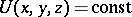. Ifis a certain force field, then the field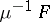hasas a potential function. If the Pfaffian equation (3) is not completely integrable, then it does not have integral surfaces but can have integral curves. If arbitrary functions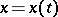,are given, then (3) will be an ordinary differential equation inand the curve,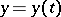,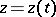will be an integral curve.

It was J. Pfaff  who posed the problem of studying equation (1) for arbitraryand of reducing the differential-formto a canonical form. Condition (4) was first obtained by L. Euler in 1755 (see ).

By a smooth change of variables any Pfaffian equation can locally be brought to the form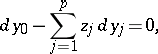(5)

whereare the new independent variables (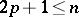,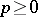). The number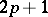is called the class of the Pfaffian equation; hereis the largest number such that the differential formof degreeis not identically zero. When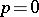the Pfaffian equation is completely integrable. The functionsare called the first integrals of the Pfaffian equation (5) and the integral manifolds of maximum possible dimension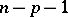are given by the equationsA Pfaffian system is a system of equations of the form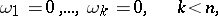(6)

whereand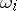are differential-forms: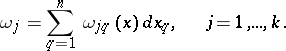The rankof the matrix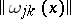is the rank of the Pfaffian system at the point. A Pfaffian system is said to be completely integrable if there is one and only one integral manifold of maximum possible dimensionthrough each point.

Frobenius' theorem: A necessary and sufficient condition for a Pfaffian system (6) of rankto be completely integrable is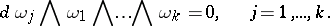The problem of integrating any finite non-linear system of partial differential equations is equivalent to the problem of integrating a certain Pfaffian system (see ).

A number of results has been obtained on the analytic theory of Pfaffian systems. A completely-integrable Pfaffian system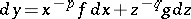ofequations has been considered, whereandare positive integers and the vector functions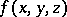,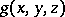are holomorphic at the point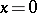,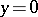,; sufficient conditions have been given for the existence of a holomorphic solution at the origin (see ); generalizations to a larger number of independent variables have also been given.

How to Cite This Entry:
Pfaffian equation. Encyclopedia of Mathematics. URL: http://encyclopediaofmath.org/index.php?title=Pfaffian_equation&oldid=18964
This article was adapted from an original article by M.V. Fedoryuk (originator), which appeared in Encyclopedia of Mathematics - ISBN 1402006098. See original article